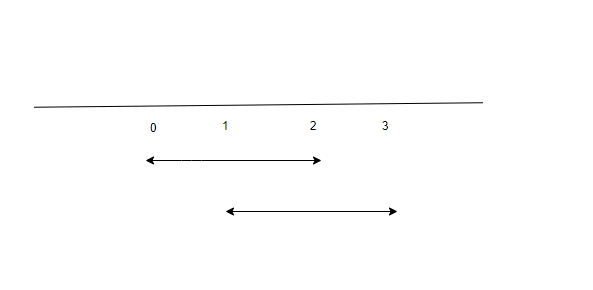New update is available. Click here to update.

# Interval List Intersection

Last Updated: 25 Jan, 2021
Difficulty: Easy

## PROBLEM STATEMENT

#### The intersection of two closed intervals is a set of real numbers that are either empty or represented as a closed interval. For example, the intersection of [0, 2] and [1, 3] is [1, 2].#### Note :

``````1. Each list of intervals is pairwise disjoint.
2. 'INTERVAL1' and 'INTERVAL2' don't contain duplicate intervals.
3. If there is no intersection present in 'INTERVAL1' and 'INTERVAL2' return an empty array/list.
``````

#### For example, if INTERVAL1 = [[0, 5], [7, 9], [10, 11]] and INTERVAL2 = [[0, 2], [3, 7], [12, 15]], then the intersection array/list will be [[0, 2], [3, 5], [7, 7]].

##### Input Format
``````The first line of input contains an integer 'T' which denotes the number of test cases or queries to be run. Then the test cases follow.

The first line of each test case contains two single-space separated integers ‘N1’ and ‘N2’, representing the length of ‘INTERVAL1 ’ and ‘INTERVAL2’ respectively.

The second line of each test case contains ‘2 * N1’ single space-separated integers denoting the intervals of the array/list INTERVAL1.

The third line of each test case contains ‘2 * N2’ single space-separated integers denoting the intervals of the array/list INTERVAL2.
``````
##### Output Format :
``````Print the list/array of intervals of the intersection of ‘INTERVAL1’ and ‘INTERVAL1’.

Print the output of each test case in a separate line.
``````
##### Note:
``````You do not need to print anything; it has already been taken care of. Just implement the given function.
``````
##### Constraints:
``````1 <= T <= 100
0 <= N1 <= 5000
0 <= N2 <= 5000
0 <= INTERVAL1[i] <=10^5
INTERVAL1[i] < INTERVAL1[i] <= 10^5
0 <= INTERVAL2[i] <=10^5
INTERVAL2[i] < INTERVAL2[i] <= 10^5

where 'T' denotes the number of test cases to be run, 'N1' and 'N2' denote the sizes of 'INTERVAL1' and 'INTERVAL2' respectively.

Time Limit: 1 second
``````## Approach 1

The main intuition behind this approach is that ‘INTERVAL1’ and ‘INTERVAL2’ are already sorted. So two cases arise:

1. If INTERVAL1 has the smallest endpoint, it can only intersect INTERVAL2. After that, we can discard INTERVAL1 since it cannot intersect with anything else.
2. If INTERVAL2 has the smallest endpoint, it can only intersect INTERVAL1. After that can discard INTERVAL2 since it cannot intersect with anything else.

Hence we can use a two-pointer algorithm.

Here is the algorithm:

1. Initialise two integer variables ‘i’ and ‘j’ that iterate over ‘INTERVAL1’ and ‘INTERVAL2’ respectively. Also initialise vector ‘intersection’ that stores the result.
1. Find the maximum of INTERVAL1[i] and INTERVAL2[j] i.e. ‘startInterval’ and minimum ofINTERVAL1[i] andINTERVAL2[j] i.e. ‘endInterval’.
2. If startInterval <= endInterval
1. Push vector {startInterval, endInterval} in ‘intersection’.
3. If INTERVAL1[i] < INTERVAL2[j]
1. i++
4. Else
1. j++
2. Finally, we return intersection.